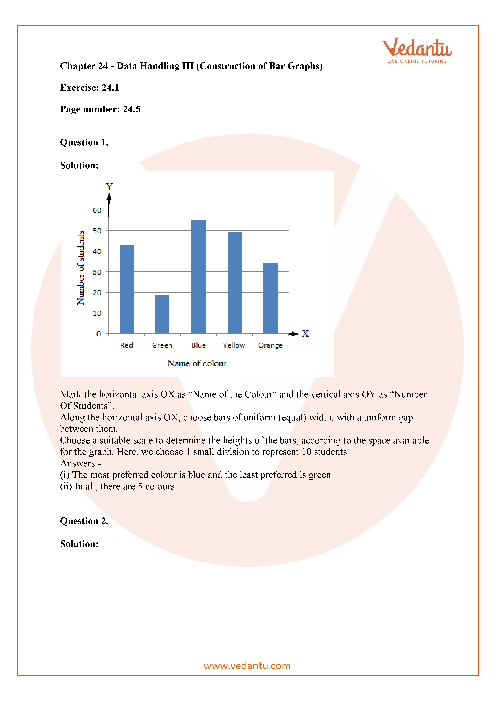# RD Sharma Class 7 Maths Solutions Chapter 24 - Data Handling-III

## RD Sharma Solutions for Class 7 Maths Chapter 24 - Data Handling III (Construction of Bar Graphs)Let's say your teacher wants to show the class comparison of student's marks in all subjects. Is it possible for her to remember every single student's marks in every subject? No way! She also requires the time to compare the marks of each student. So to do the comparison, she can use bar graphs, histograms, pie graphs, line graphs, and so on. So, in this chapter of Data Handling III students are going to learn the process of collecting, recording and displaying information in a way that is useful to others.

We discussed that Data is often graphically represented to provide a clear picture of what it represents. It may be represented by a Pictograph, a bar graph, a Double Bar Graph or Histogram. In this PDF of RD Sharma Class 7 Chapter 24 Data Handling III students will learn about Constructions of Bar Graphs which is important for Class 7 students as it tests logical thinking and presentation skills. RD Sharma Solutions for Class 7 Maths Chapter 24 will help students to improve their thinking skills and presentation skills and also to score good marks in their board exams.

Students can download a free PDF of RD Sharma Class 7 Data Handling 3 from the Vedantu platform which provides the content to all RD Sharma Chapters and classes according to the NCERT curriculum.

Do you need help with your Homework? Are you preparing for Exams?
Study without Internet (Offline)## Class 7 RD Sharma Textbook Solutions Chapter 24 - Data Handling III (Construction of Bar Graphs)

### RD Sharma Solutions for Class 7 Maths Chapter 24

The RD Sharma Solutions for Class 7 Maths Chapter 24 are prepared by the Vedantu experts who have a lot of experience in teaching Data handling chapters. Students can learn how to draw a bar graph and a double bar graph for the given data by reading this chapter. Here are the RD Sharma Class 7 Solutions, which provide the answers to all of the questions in this chapter. All the solutions are according to the NCERT curriculum. Experts have taken a lot of interest to provide step-by-step solutions to all important questions so that students can get their doubts cleared by going through the solutions. A lot of exercises and practise problems in RD Sharma Class 7 Data Handling 3 are also provided so that the students can practice before their exams and score good marks. Let's take a closer look at some of the concepts covered in this chapter.

• Definition and meaning of bar graphs

• Types of Bar Graphs

• Properties of Bar Graphs

• Steps to construct bar graphs

### Tips to Prepare for Exams Using RD Sharma Solutions for Class 7 Maths Chapter 24

Data handling is one of the important chapters in Class 7 Maths which has a higher weightage in board exams. So these tips will help students to secure good marks in their exams.

• When collecting the data try to sort those in some order rather than just collecting randomly as this will help to present the data in quicker time in charts or tables.

• Read the questions carefully before answering, sometimes the question says to present the data in graphs or charts. If we are not clear with graphs and charts then we may end up with the wrong answers.

• Try using geometrical tools while plotting graphs and charts so that the answer looks clean and tidy which increases the presentation skills.

### Conclusion

This chapter introduces students to the idea of bar graphs and shows them how to create them in a step-by-step manner. The best way to make data more visually appealing is to view it in a bar graph format. Data can be visualised using bar graphs, allowing students to understand concepts more quickly and effectively. Two or more properties of a given data are all that is needed to display data in the form of bar graphs. Students will learn about the construction of bar graphs, double bar graphs, and other major concepts in this chapter. Also, students will find it easy when studying using RD Sharma Solutions for Class 7 Maths Chapter 24 solutions prepared by Vedantu as the solutions are explained in a step by step manner. The experts have done a lot of research on the solutions so that students will enjoy reading the RD Sharma solutions. The free PDF also provides wide varieties of exercise problems for students to prepare and improve their subject knowledge.

1. What is data handling?

Data handling is the method of ensuring that research information before and after the completion of a research project is preserved, archived or disposed of in a safe and secure manner. Proper preparation for data handling will also result in data storage, retrieval, and disposal is effective and economical.

2. What Properties of Bar Graphs?

Bar graph is the most basic of the graphs. It is a graphical representation of data that uses rectangular bars or columns of equal width to display the data. It is the most basic form of data representation. Here are a few properties of Bar Graphs:

• In a bar graph, each bar or column is the same width.

• The base of all bars will be the same.

• The data value is represented by the height of the bar.

• Each bar has the same distance between them.

3. Why do I have to study RD Sharma Solutions for Class 7 Maths Chapter 24?

Data handling is one of the important chapters for Class 7 students which has a lot of weightage in their final exams. The RD Sharma Solutions for Class 7 Maths Chapter 24 are prepared by the Vedantu experts to provide a top-notch solution on data handling so that students ace their exams.SHARETWEETSHARESUBSCRIBE